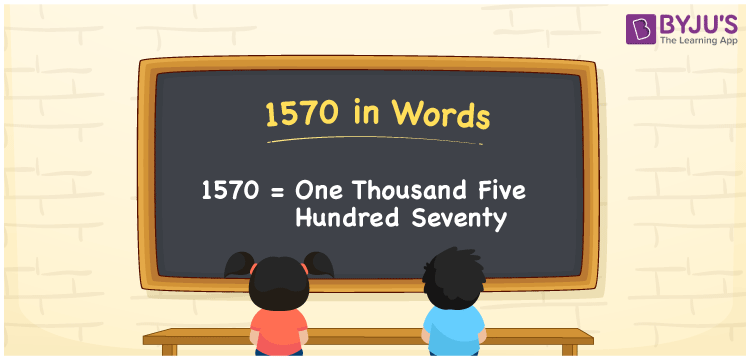# 1570 in Words

1570 in words is written as One Thousand Five Hundred Seventy. In both the International System of Numerals and the Indian System of Numerals, 1570 is written as One Thousand Five Hundred Seventy.

We often use 1570 as “1570 tickets have been sold”. 1570 represents a quantity, “how much of something” or “how many of something”. Hence, the number 1570 is a Cardinal Number.

 1570 in Words One Thousand Five Hundred Seventy One Thousand Five Hundred Seventy in Number 1570

## 1570 in English Words

1570 in English words is read as “One Thousand Five Hundred Seventy.”## How to Write 1570 in Words?

To write 1570 in words, we shall use the place value chart. In the place value chart, put 1 in the thousands, 5 in the hundreds, 7 in the tens and 0 in the ones, respectively. Let us make a place value chart to write the number 1570 in words.

 Thousands Hundreds Tens Ones 1 5 7 0

Thus, we can write the expanded form as:

1 × Thousand + 5 × Hundred + 7 × Ten + 0 × One

= 1 × 1000 + 5 × 100 + 7 × 10 + 0 × 1

= 1000 + 500 + 70 + 0

= 1570

= One Thousand Five Hundred Seventy.

1570 is a natural number between 1569 and 1571.

1570 in words – One Thousand Five Hundred Seventy

• Is 1570 an odd number? – No
• Is 1570 an even number? – Yes
• Is 1570 a perfect square number? – No
• Is 1570 a perfect cube number? – No
• Is 1570 a prime number? – No
• Is 1570 a composite number? – Yes

## Frequently Asked Questions on 1570 in Words

Q1

### How to write 1570 in words?

1570 in words is written as One thousand five hundred seventy.
Q2

### How to write 1570 in words in the International and Indian System of Numerals?

In both, the system of numerals, 1570 in words, is written as One thousand five hundred seventy.
Q3

### How to write 1570 in a place value chart?

In the place value chart, write 1 in the thousands, 5 in the hundreds, 7 in the tens and 0 in the ones, respectively.
Q4

### How to write 1570 on a Cheque?

We generally write 1570 in words on a cheque as “One Thousand Five Hundred Seventy”.
Q5

### What is the value of 1570-60? Write it in words.

1570-60=1510, which can be written as “One Thousand Five Hundred Ten”.